## 10 Questions MCQ Test Mathematics Olympiad Class 7 | Olympiad Test: Decimals -2

Description
Attempt Olympiad Test: Decimals -2 | 10 questions in 20 minutes | Mock test for Class 7 preparation | Free important questions MCQ to study Mathematics Olympiad Class 7 for Class 7 Exam | Download free PDF with solutions
QUESTION: 1

### What is the quotient if 0.0018 is divided by 0.09?

Solution: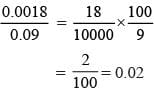QUESTION: 2

### A bowler took 17 wickets for 331 runs. What is the average score per wicket?

Solution:

Average score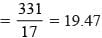QUESTION: 3

### In a school Rs 18400 is distributed equally among student of class VII. If each student gets Rs 287.5. How many students are there in class VII?

Solution:

Number of students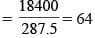QUESTION: 4

What should be subtracted from 0.103 to get 0.079?

Solution:

0.103–0.079 = 0.024

QUESTION: 5

What should be added to 5.067 to get 7.003?

Solution:

7.003–5.067 = 1.936

QUESTION: 6

The total weight of some bags of cement is 3024.80 kg. If each bag weighs 39.8 kg how many bags are there?

Solution:

No. of cement bags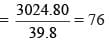QUESTION: 7

Find the difference of 9 and 7.069.

Solution:

9 – 7.069 = 1.931

QUESTION: 8

If the sum of 7.6, 0.76 and 0.716 is subtracted from 19.07, what is the result?

Solution:

19.07 – (7.6 + 0.76 + 0.716)
= 19.07 – 9.076 = 9.994

QUESTION: 9

Meena cuts 56 cm cloth into pieces of 2.24 m each. How many pieces does she get?

Solution:

No. of pieces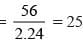QUESTION: 10

If 69 buckets of equal capacity can be filled with 586.5 litres of water. What is the capacity of each bucket ?

Solution:

Capacity of each bucket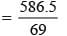= 8.5 litresUse Code STAYHOME200 and get INR 200 additional OFF Use Coupon Code